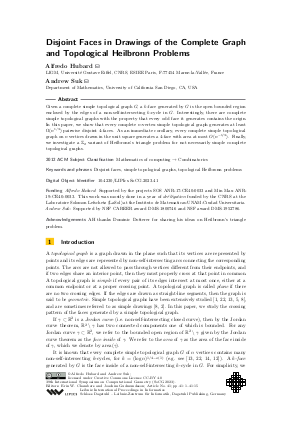Document# Disjoint Faces in Drawings of the Complete Graph and Topological Heilbronn Problems

### Authors Alfredo Hubard, Andrew Suk## File

LIPIcs.SoCG.2023.41.pdf
• Filesize: 0.92 MB
• 15 pages

## Acknowledgements

AH thanks Dominic Dotterer for sharing his ideas on Heilbronn’s triangle problem.

## Cite As

Alfredo Hubard and Andrew Suk. Disjoint Faces in Drawings of the Complete Graph and Topological Heilbronn Problems. In 39th International Symposium on Computational Geometry (SoCG 2023). Leibniz International Proceedings in Informatics (LIPIcs), Volume 258, pp. 41:1-41:15, Schloss Dagstuhl - Leibniz-Zentrum für Informatik (2023)
https://doi.org/10.4230/LIPIcs.SoCG.2023.41

## Abstract

Given a complete simple topological graph G, a k-face generated by G is the open bounded region enclosed by the edges of a non-self-intersecting k-cycle in G. Interestingly, there are complete simple topological graphs with the property that every odd face it generates contains the origin. In this paper, we show that every complete n-vertex simple topological graph generates at least Ω(n^{1/3}) pairwise disjoint 4-faces. As an immediate corollary, every complete simple topological graph on n vertices drawn in the unit square generates a 4-face with area at most O(n^{-1/3}). Finally, we investigate a ℤ₂ variant of Heilbronn’s triangle problem for not necessarily simple complete topological graphs.

## Subject Classification

##### ACM Subject Classification
• Mathematics of computing → Combinatorics
##### Keywords
• Disjoint faces
• simple topological graphs
• topological Heilbronn problems

## Metrics

• Access Statistics
• Total Accesses (updated on a weekly basis)
0

## References

1. O. Aichholzer, A. García, J. Tejel, B. Vogtenhuber, and A. Weinberger. Twisted ways to find plane structures in simple drawings of complete graphs. In Xavier Goaoc and Michael Kerber, editors, 38th International Symposium on Computational Geometry, SoCG 2022, June 7-10, 2022, Berlin, Germany, volume 224 of LIPIcs, pages 5:1-5:18. Schloss Dagstuhl - Leibniz-Zentrum für Informatik, 2022.2. A. Arroyo, M. Derka, and I. Parada. Extending simple drawings. In 27th International Symposium on Graph Drawing and Network Visualization (GD), volume 11904 of Lecture Notes in Computer Science, pages 230-243. Springer, 2019. URL: https://doi.org/10.1007/978-3-030-35802-0_18.
3. R. Courant and H. Robbins. What is Mathematics? Oxford University Press, 1941.4. P. Erdos. Problems and results in combinatorial geometry. Annals of the New York Academy of Sciences, 440(1):1-11, 1985.5. R. Fulek and A.J. Ruiz-Vargas. Topological graphs: empty triangles and disjoint matchings. In Proceedings of the 29th Annual Symposium on Computational Geometry (SoCG’13), pages 259-266, 2013.6. V. Gullemin and A. Pollock. Differentiable Geometry. American Mathematical Society. Chelsea Publishing, 1974.7. H. Harborth and I. Mengersen. Drawings of the complete graph with maximum number of crossings. Congr. Numer., 88:225-228, 1992.8. M. Hoffmann, CH. Liu, M.M. Reddy, and C.D. Tóth. Simple topological drawings of k-planar graphs. In Graph Drawing and Network Visualization, volume 12590 of Lecture Notes in Computer Science. Springer, 2020.9. J. Komlós, J. Pintz, and E. Szemerédi. On Heilbronn’s triangle problem. J. London Math. Soc., 24:385-396, 1981.10. J. Komlós, J. Pintz, and E. Szemerédi. A lower bound for Heilbronn’s problem. J. London Math. Soc., 25:13-24, 1982.11. H. Lefmann. Distributions of points in the unit square and large k-gons. European J. Combin., 29:946-965, 2008.12. A. Marcus and G. Tardos. Intersection reverse sequences and geometric applications. J. Comb. Theory, Ser. A, 113:675-691, 2006.13. J. Pach, J. Solymosi, and G. Tóth. Unavoidable configurations in complete topological graphs. Disc. Comput. Geom., 30:311-320, 2003.14. R. Pinchasi and R. Radoičić. On the number of edges in geometric graphs with no self-intersecting cycle of length 4. Towards a Theory of Geometric Graphs, Contemporary Mathematics (J. Pach, ed.), 342, 2004.15. V. V. Prasolov. Elements of homology theory. American Mathematical Society, Vol. 81, 2007.16. K. F. Roth. On a problem of Heilbronn. J. London Math. Soc., 26:198-204, 1951.17. K. F. Roth. On a problem of Heilbronn II. J. London Math. Soc., 25:193-212, 1972.18. K. F. Roth. On a problem of Heilbronn III. J. London Math. Soc., 25:543-549, 1972.19. K. F. Roth. Developments in Heilbronn’s triangle problem. Adv. Math., 22:364-385, 1976.20. A.J. Ruiz-Vargas. Empty triangles in complete topological graphs. Discrete Comput. Geom., 53:703-712, 2015.21. W. M. Schmid. On a problem of Heilbronn. J. London Math. Soc., 2:545-550, 1971/72.22. A. Suk and J. Zeng. Unavoidable patterns in complete simple topological graphs. Graph Drawing and Network Visualization, GD 2022, 2022.X

Feedback for Dagstuhl Publishing